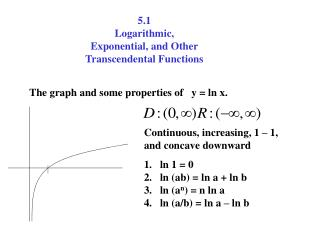DownloadDownload Presentation5.1 Logarithmic, Exponential, and Other Transcendental Functions

# 5.1 Logarithmic, Exponential, and Other Transcendental Functions

Télécharger la présentation## 5.1 Logarithmic, Exponential, and Other Transcendental Functions

- - - - - - - - - - - - - - - - - - - - - - - - - - - E N D - - - - - - - - - - - - - - - - - - - - - - - - - - -
##### Presentation Transcript

1. 5.1 Logarithmic, Exponential, and Other Transcendental Functions The graph and some properties of y = ln x. Continuous, increasing, 1 – 1, and concave downward • ln 1 = 0 • ln (ab) = ln a + ln b • ln (an) = n ln a • ln (a/b) = ln a – ln b

2. Expanding logarithmic expressions

3. first, expand it Ex. expand Ex.

4. Remember Ex.

5. Using logarithms to differentiate. First, take the ln of both sides. Ex. expand 1